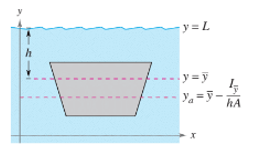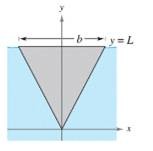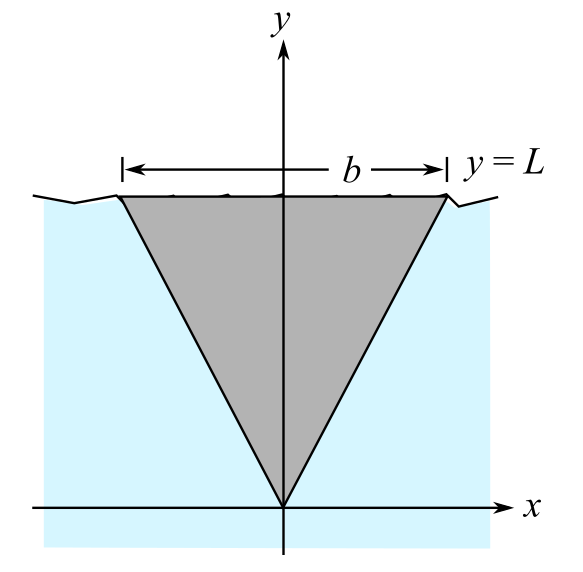Chapter 14.4, Problem 45E

Chapter
Section
Textbook Problem

Hydraulics In Exercises 43-46. determine the location of the horizontal axis y a at which a vertical gate in a dam is to be hinged so that there is no moment causing rotation under the indicated loading (see figure). The model for y a is y a = y ¯ − I y ¯ h A where y ¯ is the y-coordinate of the centroid of the gale, I y is the moment of inertia of the gate about the line y = y ¯ , h , h is the depth of the centroid below the surface, and A is the area of the gate.To determine

To calculate: The point on the horizontal axis, that is, ya, at which the vertical gate in the dam is to be hinged to make sure there is no moment that leads to rotation under the loading indicated to in the given figure,Explanation

Given:

It can be inferred from the model that ya=y¯Iy¯hA, where y¯ represents the y-coordinate of the centroid of the gate, Iy¯ represents the moment of the inertia of the gate about the line written as y=y¯, h denotes the depth of the centroid below the surface, and A is used to denote the area of the gate given in the figure.

Formula Used:

The moment of inertia is written as Iy=x1x2y1y2x2ρ(x,y)dydx, where x2 denotes the square of the distance from y-axis.

Calculation:

Have a look at the given figure,

y¯=2L3,A=bL2,h=L3 when the centroid of the triangle is written as 2L3 and the length below that point is written as L3.

About the horizontal axis, the moment of inertia is,

Iy¯=20b22LxbL(y2L3)2dydx=20b2[(y2L3)33]2LxbLd

Still sussing out bartleby?

Check out a sample textbook solution.

See a sample solution

The Solution to Your Study Problems

Bartleby provides explanations to thousands of textbook problems written by our experts, many with advanced degrees!

Get Started